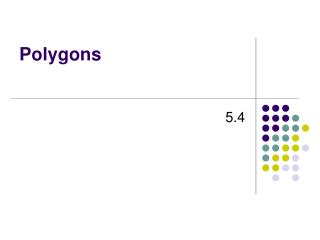DownloadDownload PresentationPolygons

# Polygons

Download Presentation## Polygons

- - - - - - - - - - - - - - - - - - - - - - - - - - - E N D - - - - - - - - - - - - - - - - - - - - - - - - - - -
##### Presentation Transcript

1. Polygons 5.4 Pre-Algebra

2. Warm Up 1. How many sides does a hexagon have? 2. How many sides does a pentagon have? 3. How many angles does an octagon have? 4. Evaluate (n – 2)180 for n = 7. 6 5 8 900

3. Learn to classify and find angles in polygons.

4. Vocabulary polygon regular polygon trapezoid parallelogram rectangle rhombus square

5. The cross section of a brilliant-cut diamond is a pentagon. The most beautiful and valuable diamonds have precisely cut angles that maximize the amount of light they reflect. A polygon is a closed plane figure formed by three or more segments. A polygon is named by the number of its sides.

6. Triangle 3 Quadrilateral 4 Pentagon 5 Hexagon 6 Heptagon 7 Octagon 8 n-gon n Polygon Number of Sides

7. Example: Finding Sums of the Angle Measures in Polygons A. Find the sum of the angle measures in a hexagon. Divide the figure into triangles. 4 triangles 4 • 180° = 720°

8. Example: Finding Sums of the Angle Measures in Polygons Continued B. Find the sum of the angle measures in a octagon. Divide the figure into triangles. 6 triangles 6 • 180° = 1080°

9. Try This A. Find the sum of the angle measures in a hexagon. Divide the figure into triangles. 4 triangles 4 • 180° = 720°

10. Try This B. Find the sum of the angle measures in a heptagon. Divide the figure into triangles. 5 triangles 5 • 180° = 900°

11. The pattern is that the number of triangles is always 2 less than the number of sides. So an n-gon can be divided into n – 2 triangles. The sum of the angle measures of any n-gon is 180°(n – 2). All the sides and angles of a regular polygon have equal measures.

12. 6x 6 720°6 = Example: Finding the Measure of Each Angle in a Regular Polygon Find the angle measures in the regular polygon. 6 congruent angles 6x = 180°(6 – 2) 6x = 180°(4) 6x = 720° x = 120°

13. 4y 4 360°4 = Example: Finding the Measure of Each Angle in a Regular Polygon Find the angle measures in the regular polygon. 4 congruent angles 4y = 180°(4 – 2) 4y = 180°(2) 4y = 360° y = 90°

14. 5a 5 540° 5 = Try This Find the angle measures in the regular polygon. 5 congruent angles 5a = 180°(5 – 2) a° 5a = 180°(3) a° a° 5a = 540° a° a° a = 108°

15. b° b° b° b° b° 8b 8 1080° 8 = b° b° Try This Find the angle measures in the regular polygon. 8 congruent angles 8b = 180°(8 – 2) 8b = 180°(6) 8b = 1080° b = 135°

16. Example: Classifying Quadrilaterals Give all the names that apply to the figure. quadrilateral Four-sided polygon parallelogram 2 pairs of parallel sides rectangle 4 right angles rhombus 4 congruent sides square 4 congruent sides and 4 right angles

17. Example: Classifying Quadrilaterals Continued Give all the names that apply to the figure. quadrilateral Four-sided polygon parallelogram 2 pairs of parallel sides rhombus 4 congruent sides

18. Try This Give all the names that apply to the figure. A. quadrilateral Four-sided polygon parallelogram 2 pairs of parallel sides rectangle 4 right angles

19. Try This Give all the names that apply to the figure. B. quadrilateral Four-sided polygon

20. Lesson Quiz: Part 1 1. Find the sum of the angle measures in a quadrilateral. 2. Find the sum of the angle measures in a hexagon. 3. Find the measure of each angle in a regular octagon. 360° 720° 135°

21. Lesson Quiz: Part 2 4. Write all of the names that apply to the figure below. quadrilateral, rhombus, parallelogram